Reach Us+447482877761
Physical Properties of Pure and Nano Ag Doped Liquid Crystalline Compounds Containing 1,3,4-Oxadizole Unit | OMICS International
Chemical Sciences Journal
All submissions of the EM system will be redirected to Online Manuscript Submission System. Authors are requested to submit articles directly to Online Manuscript Submission System of respective journal.

# Physical Properties of Pure and Nano Ag Doped Liquid Crystalline Compounds Containing 1,3,4-Oxadizole Unit

Naser JA1, Himdan TA1, Latif IA1, Mohammed YI1 and Al-Dujaili AH2*

1Department of Chemistry, College of Education for Pure Sciences, Ibn Al-Hiatham, University of Baghdad, Baghdad, Iraq

2Department of Chemistry, Faculty of Science, University of Jordan, Amman, Jordan

Corresponding Author:
Al-Dujaili AH
Department of Chemistry
Faculty of Science
University of Jordan
Amman, Jordan
E-mail: [email protected]

Received Date: August 22, 2015; Accepted Date: August 24, 2015; Published Date: September 01, 2015

Citation: Naser JA, Himdan TA, Latif IA, Mohammed YI, Al-Dujaili AH (2015) Physical Properties of Pure and Nano Ag Doped Liquid Crystalline Compounds Containing 1,3,4-Oxadizole Unit. Chem Sci J 6:105. doi:10.4172/2150-3494.1000105

Copyright: © 2015 Naser JA, et al. This is an open-access article distributed under the terms of the Creative Commons Attribution License, which permits unrestricted use, distribution, and reproduction in any medium, provided the original author and source are credited.

Visit for more related articles at Chemical Sciences Journal

#### Abstract

Dielectric properties and other physical properties such as electrical conductivity (AC) and relaxation time or activation energy have been studied for two systems pure LC [V]6,6, [V]7,6, [V]8,6, [V]6,7, [V]7,7 and [V]8,7 and their doped with silver nanoparticles. The results show the increasing in real dielectric permittivity έ with increasing length terminal chain. So the real dielectric permittivity increasing with raising temperature. To compare between the values of (400) Hz and (4000) Hz we observe these values at the low frequency are larger than that in high frequency. Generally, one can see that the doping of Ag nano particles effectively reduced the permittivity of the LC materials with its large electric dipole moment. The electrical conductivity σ value for pure LC samples in general increases with increasing temperature. So we observed increasing of electrical conductivity values at high frequency. The time scale is discussed in terms of the Arrhenius plot. Generally, with increasing the temperature the time period that spends by molecules at the transition state will increase. The activation energy Ea values show the increase in the activation energy to the doped systems.

#### Keywords

Liquid crystals; Dielectric permittivity; Electrical conductivity; Relaxation time; Activation energy; Doped liquid crystals; Silver nanoparticles

#### Introduction

The importance of liquid crystals lies in their extensive use in display devices, it is well known that liquid crystals are very sensitive to an electrical field. This property allows their applications to displays and other optical devices technology [1-5]. However the use of liquid crystals in different devises depends upon various properties owned by them like order parameter, dielectric permittivity, dielectric anisotropy, birefringent behavior, optical transmittance, elastic constants etc. . Most applications of liquid crystals depend upon the possibilities of changing orientation of the liquid crystal molecules by the applied electric fields, which in turn depends on the visco-elastic and electrical properties [7-9].

A particular application of liquid crystals requires a particular set of parameters of the liquid crystal in required range. The dielectric studies have been very useful as they provide important information regarding molecular structure, molecular dynamics, and type of molecular interactions in the sample . The nature of nanomaterials may be conducting, semiconducting, dielectric, insulating, organic, inorganic and is available in different sizes and shapes like spherical, wires, tubes, rods etc. [10,11].

Liquid crystals are very important materials because of their scientific and technological importance, but a single liquid crystal material cannot fulfill all the requirements for a practical device. Therefore, the different mixtures of liquid crystals and their doped samples have gained growing interest. These doped samples have emerging prospects regarding their applications in information processing .

In the present paper, our aim is to study some physical properties such as dielectric permittivity, electrical conductivity and relaxation time or activation energy for two systems pure LC [V]6,6, [V]7,6, [V]8,6, [V]6,7, [V]7,7 and [V]8,7 and their doped with silver nanoparticles. The general structure of compounds have been studied is shown in (Figure 1). All of the compounds in both two series [V]m,6 and [V]m,7 show mesomorphic properties. All members of those two series exhibit an enantiotropic dimorphism smectic A (SmA), smectic C (SmC) and/or nematic (N) phases.

#### Experimental

Materials

Liquid crystalline compounds which studying were synthesized and published in our previous work . Continually, to improve the physical study, Silver nanoparticles were prepared by reduction method and identified by mostly analysis XRD, SEM and AFM. From all of these techniques the particle size of nano seemed less than 50 nm.

Instrumentation

Dielectric permittivity measurements:

The dielectric behavior of the materials has been studied by using an instrument to measure dielectric constant LCR meter (hp- 4274A) multi-frequency, based heating device hot stage polarizing microscope. The dielectric parameters have been measured as a function of temperature range 90°C-160°C at 400 Hz and 4000 Hz. The samples heated to reach isotropic phase, then cooled the parameter of dielectric constant and resistance have been recorded each 10°C.

Preparation of cells:

Two similar cells having active areas of 1 cm2 were prepared by using transparent and highly conducting ITO (indium tin oxide) coated optically flat glass substrates used as electrodes. These electrodes give a base to the LC sample to align. Planar alignment is obtained by treating with both an adhesion promoter and a polymer (polyimide PI2555) and then rubbed unidirectionally with a velvet cloth. The thickness of the cell was maintained at (12 μm) by means of mylar spacers. The correct and proper alignment of the LC molecules is extremely important for precise measurement of electrical properties, which in turn influences the dielectric parameters and thus plays an extremely important role in molecular geometry.

Preparation of samples:

Sandwich cells have been heated on hotplate softly after that materials impacted by capillary action. That is for pure LC samples, while for LC doping silver nano particle samples, take 0.1 gm of LC then mixed with 0.025 gm from silver nano particle in 15 mL of benzene as a solvent. The solution translated to ultrasonic instrument under 6 force to destroy aggregations, let solvent to evaporate then the doping systems was collected and impacted in the same way.

Preparation of silver nano particles:

Silver nitrate AgNO3 and trisodium citrate C6H5O7Na3 of analytical grade purity were used as starting materials. The silver colloid was prepared by using chemical reduction method . All solutions of reacting materials were prepared in distilled water. In typical experiment 250 mL of 0.01 M AgNO3 was heated to boiling. To this solution 25 mL of 5% trisodium citrate was added drop by drop. During the process solution was mixed vigorously and heated until color’s change is evident (pale yellow). Then it was removed from the heating element and stirred until cooled to room temperature, Ag nano particles were collected and washed many times . Chemical equation of the reaction could be express as follows [16,17]

4Ag+ +C6H5O7Na3 + 2H2O → 4Ag0 + C6H5O7H3 + 3Na++ H++ O2↑

Characterization of silver nano particles:

Characterization of nanoparticles is important to understand and control nanoparticles synthesis and applications. Characterization is performed using a variety of different techniques such as powder X-ray diffractometry (XRD), scanning electron microscopy (SEM) and atomic force microscopy (AFM). These techniques are used for determination of different parameters such as particle size, shape and crystallinity. For instance, the morphology and particle size could be determined by SEM and AFM. The advantage of AFM over traditional microscopes such as SEM is that AFM measures three-dimensional images so that particle height and volume can be calculated. Moreover, X-ray diffraction is used for the determination of crystallinity .

XRD analysis:

In Figure 2 shows the XRD pattern of powder silver nano particle. There is presence of three largest peaks at 2ɵ values 38.1203, 44.2895 and 64.4542. Thus, the XRD spectrum confirmed the crystalline structure of silver nano particles. All the peaks in XRD pattern can be readily indexed to a face-centered cubic structure of silver. However, the diffraction peaks are broad which indicating that the crystallite size is very small. The nano particle size (L) has been calculated by Deby Scherrer’s formula .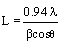(1)

Where λ is wave length 0.167 A0 of X-rays used, β is broadening of diffraction line measured at half of its maximum intensity (in radian) and ɵ is Bragg’s diffraction angle (in degree). The crystallite size of silver nano particles have been found to be 49.18 nm, 33.88 nm and 19.41 nm.

SEM analysis:

Scanning electron microscopy has provided further insight into the morphology and size details of the synthesized silver nano particles are shown in (Figure 3). The large aggregation was found attributed to not using a disperser in the techniques

AFM analysis:

Atomic force microscopy indicates particles and distribution average size, which was less than 50 nm as shown in (Figure 4).

#### Results and Discussion

Dielectric permittivity properties

Measured quantities were the capacitance (Cx) and resistance (R) of the specimen, so real dielectric permittivity (έ) and imaginary dielectric permittivity ( ε'' ) were calculated as follow equations [19-21].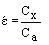(2)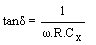(3)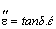(4)

where Ca is the capacitance of the cell with air which is equal to (0.0885)A ⁄ t; A is the area of the electrode in m2, which equal 0.001 m2 and t is the thickness of the specimen in m, which equal 12 μm. Cx is the electrostatic capacity of the specimen in pf, R is the resistance of the specimen and ù = 2ðf , f; is the applied frequency. In the present investigation, two types of cells were prepared, one for pure LC material and another one with Ag nanoparticles doped LC material, for comparative study. In Figures 5 and 6 shows the temperature dependence of real dielectric permittivity έ for the pure liquid crystalline samples at frequency 400 Hz and 4000 Hz. While Figures 7 and 8 shows the temperature dependence of real dielectric permittivity έ for pure liquid crystalline and nano Ag doped liquid crystalline [V]8,6 and [V]8,7 samples (as a representative examples) at frequencies 400 Hz and 4000 Hz, the characteristic features of these measurements can be listed as follow:

Figure 5: Variation of real dielectric permittivity with range of temperatures at 400 and 4000 Hz frequency for compounds [V]6,6, [V]7,6 and [V]8,6.

Figure 6: Variation of real dielectric permittivity with range of temperatures at 400 Hz frequency for compounds [V]6,7, [V]7,7 and [V]8,7.

Figure 7: Variation of real dielectric permittivity with range of temperatures at 400 and 4000 Hz frequency for doped and undoped compound [V]8,6.

Figure 8: Variation of real dielectric permittivity with range of temperatures at 400 and 4000 Hz frequency for doped and undoped compound [V]8,7.

1. In all figures έ increases with increasing temperature at both frequencies.

2. In the both series [V]m,6 and [V]m,7 at all temperature range the value of έ at 400 Hz is larger than that at 4000 Hz.

3. There is an increase in έ value with increasing length of the alkyl side chain in the both frequencies for all pure samples.

4. The value of έ for [V]m,6 is larger than that of [V]m,7.

5. Addition of Ag nano particles acts to decrease value considerably.

In series [V]m,6 there is a great change in thermal coefficient dέ/dT between doped and undoped samples, but in [V]m,7 series this coefficient is approximately in doped and undoped samples.

There is no considerable change in dέ/dT by transforming from solid crystals to liquid crystalline mesophase then to isotropic phase.

The first point in the above list can be described as follows: one of the meanings of real dielectric permittivity is the tendency of the permanent or induced dipole moment of the molecular system to alignment with applied electrical field. From this view point we can describe the real dielectric permittivity behavior with temperature as follow, at low temperatures when the measomorphic compounds at solid state the thermal motion of the molecules cannot arrange with applied field but with increasing temperature during the transition to mesomorphic phase, the increasing thermal motion of the molecules liberate these molecules and give their more ability to engage with varying electrical field.

To compare between the values of έ at 400 Hz and 4000 Hz we observe these values at the low frequency are larger than that in high frequency, this indicate that thermal motion of molecules can incorporate with applied electrical field at low frequency 400 Hz, while at higher frequency 4000 Hz this motion cannot follow the rate of varying field, as shown in previous figures. The increasing in real dielectric permittivity έ with increasing length terminal chain is due to the hydrophobic behaviors (less polarizability), interpretation of the relation of long the side chain of the terminal groups and the value of according to the law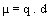(5)

Where, μ is the electric dipole moment. In the simple case of two point charges, one with charge +q and the other one with charge –q while d is the displacement vector pointing from the negative charge to the positive charge. The increase of the distance between opposite charges will increase the dipole moment value (induced or permanent), so that έ will increase.

The dropping of έ value in the presence of Ag nanoparticles is explained in the light of that dielectric medium have dipole moment and orientation polarization (atomic polarization and electronic polarization) , that the space charge density is higher at lower frequency in the bulk as well as near the surfaces. With increasing the frequency the space charge density is reduced in the bulk as well as near the surfaces. Therefore, at lower frequency the space charge effect is dominant. When nano particles were doped into the LC system, the orientation of nano particle was such that the dipole moment of nano particles are opposing the dipole moment of LC molecule and that dielectric constant decreases for nano doped systems . Same figures show the relationship between the dielectric permittivity and temperature for pure LC and Ag nano particles doped LC system in a 400 Hz and 4000 Hz frequency. The dielectric permittivity has increased drastically with increases temperature. We can see from figures that there is very large difference in the dielectric permittivity for pure LC and Ag nano particles doped LC systems. It seems to indicate that nano particles are broken the symmetry of LC molecule, the dipole moment of nano particles are anti-parallel direction of the dipole moment of LC molecule . Therefore, we can see that the doping of Ag nano particles effectively reduced the dielectric permittivity of the LC materials with its large electric dipole moment.

Imaginary dielectric permittivity

The real dielectric permittivity property of the medium is a parameter of how much energy from an external electric field is stored in a material, while imaginary dielectric permittivity or the loss angle is a parameter of the lost energy by the field when this external field is reflect .

The loss tangent or tan δ is defined as a ratio of the imaginary part of the dielectric permittivity to the real part. The imaginary dielectric permittivity is a measure of lost energy of the oscillating electrical field that happened when electrical moments inside the insulator go to follow the oscillating applied electrical field . As we see previously the increasing of temperature act to enable the molecular moments to follow the applied electrical field, on the other hand increasing of temperature act also to increase the random motion of the molecules as well, so there will be a quantity of electrical field energy consumed to organize the random directed molecular electrical moments in the direction of the applied field. In Figures 9 and 10 show the temperature dependence of imaginary dielectric permittivity or dielectric loss factor ε'' of pure liquid crystalline and nano Ag doped liquid crystalline [V]6,6 and [V]7,7 (as a representative examples) samples at 400 Hz and 4000 Hz. These behaviors can be summarized as:

Figure 9: Variation of imaginary dielectric permittivity with range of temperatures at 400 and 4000 Hz frequency for doped and undoped compound [V]6,6.

Figure 10: Variation of imaginary dielectric permittivity with range of temperatures at 400 and 4000 Hz frequency for doped and undoped compound [V]7,7.

1. For pure samples ε'' increases with increasing temperature but in a non-linear manner where is there are points of discontinuity of thermal coefficient dέ/dT with increasing temperature.

2. ε'' values at 400 Hz are greater than that at 4000 Hz except for [V]m,7 series where its values at 400 Hz overlap in an oscillating form with that at 4000 Hz.

3. With increasing length of the side chain of both series the thermal coefficient dέ/dT decreases while by comparison between the two series the value of ε'' for [V]m,6 series is greater than that of [V]m,7 series.

4. For doped samples the values of ε'' are smaller than that for pure samples and also they have a low value of dέ/dT relative with pure samples. With increasing length of the side chain there is an apparent decrease of ε'' values in both series and finally ε'' values for [V]m,6 series are larger than that of [V]m,7 series.

The non-linear response of ε'' with temperature indicates that ε'' is more sensitive to the phase transformations than ε'' where the discontinuity points on the curve belong to the transition temperatures of the LC material this sensitivity may be due to nature of the lost energy by the field which related to the entropy of transformation. Generally, with increasing temperature at both frequencies observe increasing of imaginary dielectric constant values with increasing temperature as a result to increase the random orientation of molecular moments with increasing temperatures, which act to disperse or consume more lost energy to orient these moments with applied external field. This case is clear in pure LC systems, while in doped Ag nano LC systems, the thermal coefficient dέ/dT of this process is smaller than that in pure samples. The reason of this due to the sharp reduction of the number of molecular moments and molecular mobility as well which caused by the addition of Ag nano particles that retract the molecular mobility through electrostatic double layer that quench the molecular dipoles of LC. These factors tend to decrease this thermal response in both states (decreasing or increasing with temperatures).

In comparison between ε'' values at 400 Hz and 4000 Hz we observe these values seems to be close to each other which does not agree with ε '' values at these different frequencies, those the έ values at 400 Hz is greater than that at 4000 Hz, this may be due to that the lost energy that expressed by ε'' is depend on the thermal energy more than its dependence on the applied external field, so we are think that ε'' is related to entropy of the system with cretin relation. However, the imaginary dielectric permittivity have slightly lower values at 4000 Hz from that 400 Hz, this due to the molecules have not ability to flow the alternating electrical field at high frequency, so the lost energy that consumed during the alternation process will be small. While at 400 Hz the molecules of the medium can alternate, the quantity of the lost energy will be larger than that in 4000 Hz.

For doped samples the values of ε'' are smaller than that for pure samples and also they have a low value of d relative with pure samples with increasing length of the side chain there is an apparent decrease of ε'' values in both series and finally ε'' values for [V]m,6 series are larger than that of [V]m,7 series.

We can explain the decreasing of imaginary dielectric permittivity in the doped systems by that the doped Ag nano particles through its charge surface which naturalize the dipole moment of LC molecules, so there will be great collapse in dielectric property of medium as result of existence of large surface area to nano particles and hence the surface charges or electrostatics double layers act as retarder quenching factors for the molecular dipole moments and therefore the over all ε'' of medium will be low. In the doped Ag nano particles systems as we see before act to restrict the mobility of molecules and quench their dipole moments, hence the lost energy by the field will be a very low at both two frequencies because of the number of dipoles that can flow the alternating field will be largely reduced. As unique case in [V]8,7, we see the imaginary dielectric permittivity has low values in both frequencies so the different is very small between 400 Hz and 400 Hz, we can conclude that the increasing in the terminal chain act to allow the molecular moments follow the alternating external field in both frequencies as well, so the value of έ and ε'' in closed to each other in pure materials.

#### Electrical conductivity

Electrical conductivity (AC) values have been calculated by following equation :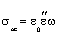(6)

where ε0 is the free space dielectric permittivity (8.85*10-12) F/m, ε'' is imaginary dielectric permittivity, ω is the angular frequency of applied electric field, which equal (2πf). In Figures 11 and 12 show the variation of σ values with the temperatures at two frequencies for doped and pure liquid crystalline [V]6,6 and [V]8,7 (as a representative examples) samples.

Figure 11: Variation of electrical conductivity with range of temperatures at 400 and 4000 Hz frequency for doped and undoped compound [V]6,6.

Figure 12: Variation of electrical conductivity with range of temperatures at 400 and 4000 Hz frequency for doped and undoped compound [V]8,7.

The characteristic features of these figures are:

1. Electrical conductivity σ increases with temperature like ε'' in a non-linear form.

2. The value of σ at 4000 Hz is greater than that at 400 Hz, the thermal coefficient of σ dσ / dT at 4000 Hz is also greater than that at 400 Hz.

3. σ and dσ / dT decrease with increasing length of alkyl side chain at both frequencies.

4. In doped samples σ is lower than that of pure samples in addition σ at 400 Hz is close in value and slope dσ / dT to the σ of doped samples at both frequencies.

The conductivity value for all samples is typical for semi-conductors . For pure LC samples in general there are an increase in σ value with increasing temperature, this is a normal situation according to Boltzman law equation (6) :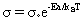(7)

Where σ° is the pre-exponential factor, Ea is the activation energy, kB is Boltzmann’s constant and T is the absolute temperature. Which indicates that materials like insulators, semi-conductors and ionic conductors the temperature acts to increase the number of charge carriers through providing these carriers with energy that required to cross the band gap between equivalent and conducting bands. The nonlinear increasing of electrical conductivity indicates its sensitivity to phase transformations that taking place to the sample with increasing temperature .

To explain the difference of σ and its thermal gradient at the two frequencies we need to focus on mechanism of charge transport, σ is depending on two factors the first one is the number of charge carriers, the second one is the mobility of these carriers. At 400 Hz thermal conductance increase with temperature but in less gradient factor than that of 4000 Hz the reason can be described by the charge mobility factor not by number of charge type, in solid state charge transferred by three mechanism the first one is the transfer from a conductance band to other neighboring band in overlapping with first one, this mechanism accurse in typical conductors. The second mechanism take place when there are a potential barrier between the conductance band and its neighboring conductance bands but this potential is small so the charges can hopping from conductance band to its neighbors. The third mechanism is a tunnel effect mechanism which is taking place when the potential barrier is greater than the charge kinetic energy. This mechanism has a very small contribution to the charge mobility . From above discussion we can conclude that the low gradient value of thermal conductivity at 400 Hz is due to the change of mobility of the charge carriers through the hopping mechanism and not by the increasing number of charge carriers which is develop through increasing temperature. From the same figures, we can observe increasing of electrical conductivity values at high frequency, this can be explained by that when field oscillation time is much smaller than the relaxation time of the substance, then the ionic atmosphere around the charge carriers cannot follow the moving charge carrier is more free in movement with oscillating field, this process is happened in both doped and pure LC systems as well.

The effect of increasing length of the side chain on σ and dσ / dT is the decreasing both of them the decrease of σ happened as a result of increasing of the hydrophobic character of the substance which reduces σ value. The decrease of dσ / dT comes from increasing the distance among conducting bands through the increasing of molecular length which act to raise the energy barrier among the conducting bands and leads to decrease the probability of crossing the charge carrier through hopping mechanism among conducting bands.

In addition to that previous explanation, we can conclude that the silver nano particles in a matrix of LC materials act as a trap to charge carries through two ways, the first one by acting of collecting the free electrons in the conducting bands that characterized its metallic nature, these nano particles of silver seems to be separated as islands which the charge transfer by them through hopping mechanism. The second way of trapping charge carriers come from the high surface area of nano systems and electro static double-layer of these surfaces which scavenge any free charge exist.

Relaxation time and activation energy

The term relaxation time means the time period starting from the formation of some kind of molecular aggregation until the disappearance of it, this term is used frequently to describe a state of statistical equilibrium of the system and the rate of the appearance of this equilibrium is related with transition probability of that system from initial equilibrium state to another equilibrium state. In the case of dielectric measurements the first equilibrium state describe the molecular configuration in absence of electrical field while the second equilibrium state describe the molecular configuration in the presence of the electrical field. In addition relaxation time is calculated from the equation (7) .

The temperature dependence of relaxation time follows Arrhenius law which is given by equation (8) [27-29]: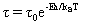(8)

Where Ea is the activation energy, τ0 is the pre-exponential factor kB is Boltzmann’s constant and T is the absolute temperature. The Arrhenius equation describes the behavior for a process from one state to another separated by a potential barrier and the height of the barrier translates the activation energy . Generally at low values of Ea or at high temperatures the relaxation time will be small because the molecules will have enough energy to cross the barrier of activation energy from initial configuration to the second configuration therefore the process will be fast or the relaxation time will be small, so the situation is reversed at high values of Ea or at low temperatures which the process will be slow and the system will need more time to transfer from configuration to other one.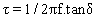(9)

In Table 1 indicates the value of activation energy calculated from the slope of the ln τ versus 1/T plot (figures not shown) that required to transforming of the molecular configuration at tow electrical field frequencies for pure liquid crystalline and Ag nano particle doped samples, this table shows the following:

Samples Ea (J/mol)400 Hz Ea (J/mol) 4000 Hz Samples Ea(J/mol) 400 Hz Ea (J/mol) 4000 Hz
[V]6,6 670.7 677.0 [V]6,6+Nano Ag 2357.9 1538.9
[V]7,6 864.7 888.7 [V]7,6+Nano Ag 3347.2 2158.8
[V]8,6 1112.4 1151.4 [V]8,6+Nano Ag 4873.7 2816.8
[V]6,7 721.9 749.4 [V]6,7+Nano Ag 1302.8 1229.6
[V]7,7 707.4 774.1 [V]7,7+Nano Ag 1674.4 1308.0
[V]8,7 697.0 897.1 [V]8,7+Nano Ag 1805.0 1520.6

Table 1: Activation energy values for pure LC and nano Ag doped samples.

1. Activation energy Ea for doped samples are greater from that for pure samples.

2. For all series at all frequencies Ea increases with increasing length of the side chain except for series [V]m,7 at 400 Hz which show an opposite behavior.

3. For pure samples Ea value at 4000 Hz is larger than that in 400 Hz, this case is reversed for doped samples where Ea value at 4000 Hz is smaller than that in 400 Hz.

The increase in the activation energy of the doped samples may be due to the restricting of the conformational motions that happened in LC terminal chain materials or reducing the mobility of these molecules through the interaction (adsorption) with the surface of nano particles.

With increasing length of the side chain we expect increasing the relaxation time that required to re-establishing an equilibrium configuration and the increase of τ value appear as increase in Ea value, the reason is due to the increase of internal degrees of freedom of the molecules and raising the probabilistic number of conformations which the LC molecule can have before reaching the optimum one that is necessary for establishing the equilibrium. For [V]m,7 series the abnormal behavior at these frequencies is belong to the same reasons that appeared by this series in previous measurements.

As a result of the harmonic response of LC molecules in the pure samples with applied electrical at 400 Hz the activation energy will be less than that at 4000 Hz because the speed period time of alternating field do not give enough time for the LC molecules to follow its alternation, therefore activation energy at high frequency 4000 Hz will be less than that at low frequency 400 Hz, the LC molecules are in harmonic movement with applied field at 400 Hz but this motion are loaded with bulky Ag nano particles so its require more energy to confirm this harmonic motion this leads to the LC molecules which do not response well with applied field at 4000 Hz need a lower energy to reach the equilibrium when the LC molecules doped with Ag nano particles.

#### References

Select your language of interest to view the total content in your interested language

### Article Usage

• Total views: 12585
• [From(publication date):
September-2015 - Aug 22, 2019]
• Breakdown by view type
• HTML page views : 8666
• PDF downloads : 3919

## Post your commentCan't read the image? click here to refresh
###### Peer Reviewed Journals

Make the best use of Scientific Research and information from our 700 + peer reviewed, Open Access Journals

International Conferences 2019-20

Meet Inspiring Speakers and Experts at our 3000+ Global Annual Meetings

Top